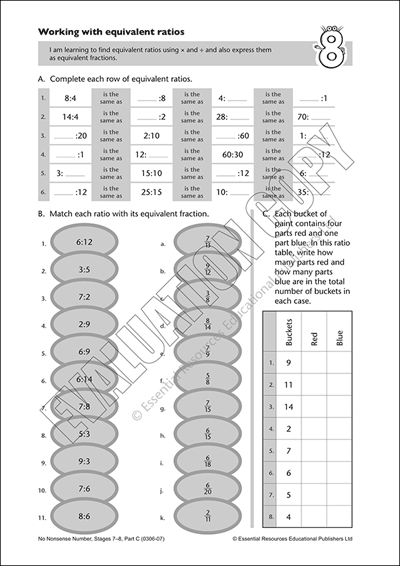# Equivalent ratios

Code: E0306-07In this worksheet students learn to find equivalent ratios using multiplication and division and also express them as equivalent fractions. Task 1 requires students to fill the gaps in a ratio table displaying four equivalent ratios per row. The following task askes students to match each given ratio with its equivalent fraction and the final activity requires students to complete a ratio table based on a word problem. All answers for these tasks are provided.

Subjects: | Resource Types: | Pages: 2 | Years: 8-9

 or more each